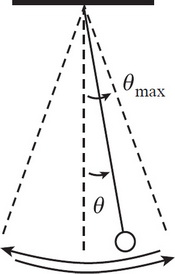# AP Physics 1 Practice Test 3

### Test Information10 questions18 minutes

1. Two spheres of net charge +5e and -6e briefly come into contact. Afterward, which of the following is a possible combination of net charges for the two spheres?

2. In which of the following situations involving a source of sound and a detector of the sound is it possible that there is NO perceived Doppler shift?

3. If L, M, and T denote the dimensions of length, mass, and time, respectively, what are the dimensions of power?

4. An object (mass = m) above the surface of the Moon (mass = m) is dropped from an altitude h equal to the Moon’s radius (R). What is the object’s impact speed?

5. When turning a corner on a bicycle, the rider leans into the turn. The frame of the bike is nearly parallel to which vector?

6.In the diagram above, a positive charge and negative charge are placed at y = -2 and y = 3, respectively. If the negative charge has a greater magnitude, then the only place of 0 net electric field would be

7. A projectile is launched with an initial velocity of magnitude a at an angle β to the horizontal. Find its maximum vertical displacement, ignoring effects due to the air.

8.A simple pendulum executes simple harmonic motion as it swings through small angles of oscillation. If θmax denotes the amplitude of the oscillations, which of the following statements is true?

9. A wire is split into 4 segments, and they have resistances of R, 2R, ½R, and 4R in that order. Which of the following sets of changes from one segment to the next could correctly account for the changes in resistance?

R→2R    2R→4R    4R→R

10. Two traveling waves of equal frequency, one of amplitude 4 cm and the other of amplitude 6 cm, superimpose in a single medium. Which of the following best describes the displacement, D, of the resultant wave?